# Exercise 2.2 Fractions and Decimals - NCERT Maths class 7

Go back to  'Fractions and Decimals'

## Chapter 2 Ex.2.2 Question 1

Which of the drawing $$(a)$$ to $$(d)$$ shows:

i) \begin{align}2 \times \frac{1}{5}\end{align}

ii) \begin{align}2 \times \frac{1}{2}\end{align}

iii) \begin{align}3 \times \frac{2}{3}\end{align}

iv) \begin{align}3 \times \frac{1}{4}\end{align}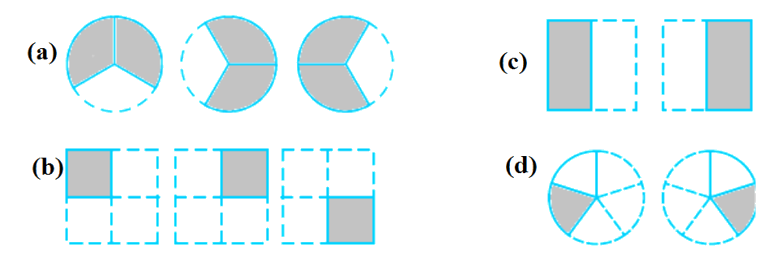### Solution

What is Known?

Fractions and Drawings

What is unknown?

Matching of fractions with shaded part of the drawings.

Reasoning:

Matching can be easily done by comparing the fractions and shaded areas.

Steps:

i)  \begin{align}2 \times \frac{1}{5}\end{align} matches with $$(d)$$ since two circles are divided in to five parts and one part of both the circles is shaded.

\begin{align} 2 \times \frac{1}{5} = \frac{1}{5} + \frac{1}{5} = \frac{2}{5} \end{align}

ii) \begin{align}2 \times \frac{1}{2}\end{align}matches with $$(b)$$ as one half of both the drawings is shaded.

\begin{align} 2 \times \frac{1}{2} = \frac{1}{2} + \frac{1}{2} = \frac{2}{2} = 1 \end{align}

iii) \begin{align}3 \times \frac{2}{3}\end{align}matches with $$(a)$$ since two third of the three circles is shaded.

\begin{align} 3 \times \frac{2}{3} = \frac{2}{3} + \frac{2}{3} + \frac{2}{3} = 3 \times \frac{2}{3} = 2 \end{align}

iv) \begin{align}3 \times \frac{1}{4}\end{align}matches with $$(c)$$ since one fourth of three squares is shaded.

\begin{align} 3 \times \frac{1}{4} = \frac{1}{4} + \frac{1}{4} + \frac{1}{4} = \frac{3}{4} \end{align}

## Chapter 2 Ex.2.2 Question 2

Some pictures $$(a)$$ to $$(c)$$ are given below. Tell which of them show:

(i) \begin{align}3 \times \frac{1}{5} = \frac{3}{5}\end{align}

(ii) \begin{align}2 \times \frac{1}{3} = \frac{2}{3}\end{align}

(iii) \begin{align}3 \times \frac{3}{4} = 2\frac{1}{4}\end{align}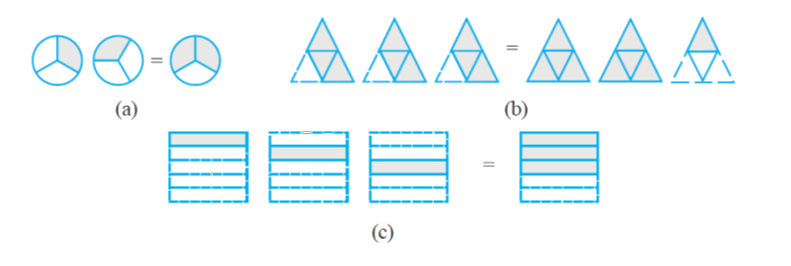### Solution

What is Known?

Fractions and pictures.

What is unknown?

Matching of fractions with shaded part of the picture.

Reasoning:

Matching can be done by comparing the fractions with the shaded areas of the pictures.

Steps:

(i) \begin{align} 3 \times \frac{1}{5} = \frac{3}{5} \end{align} matches with (c) since, \begin{align} 3 \times \frac{1}{5} = \frac{1}{5} + \frac{1}{5} + \frac{1}{5} = \frac{3}{5} \end{align}

(ii) \begin{align} 2 \times \frac{1}{3} = \frac{2}{3} \end{align}  matches with (a) Since \begin{align} 2 \times \frac{1}{3} = \frac{1}{3} + \frac{1}{3} = \frac{2}{3} \end{align}

(iii) \begin{align} 3 \times \frac{3}{4} = 2\frac{1}{4} \end{align} matches with (b) Since, \begin{align} 3 \times \frac{3}{4} = \frac{3}{4} + \frac{3}{4} + \frac{3}{4} = 2\frac{1}{4} \end{align}

## Chapter 2 Ex.2.2 Question 3

Multiply and reduce to lowest form and convert into a mixed fraction: -

(i)\begin{align}\,\,7 \times \frac{3}{5}\end{align}

(ii) \begin{align}4 \times \frac{1}{3}\end{align}

(iii)\begin{align}\,\,2 \times \frac{6}{7}\end{align}

(iv)\begin{align}\,\,5 \times \frac{2}{9}\end{align}

(v)\begin{align}4 \times \frac{2}{3}\end{align}

(vi)\begin{align}\,\,6 \times \frac{5}{2}\end{align}

(vii)\begin{align}\,\,11 \times \frac{4}{7}\end{align}

(viii)\begin{align}20 \times \frac{4}{5}\end{align}

(ix)\begin{align}\,\,13 \times \frac{1}{3}\end{align}

(x)\begin{align}15 \times \frac{3}{5}\end{align}

### Solution

What is Known?

Fractions multiplied with some numbers.

What is unknown?

Lowest form and mixed form of the fractions

Reasoning:

Multiply the numerator with the given number to get improper fractions, Then convert the improper fractions in to mixed fractions.

Steps:

(i) \begin{align} 7 \times \frac{3}{5} = \frac{{7 \times 3}}{5} = \frac{{21}}{5}\end{align}(improper fraction as numerator is greater than the denominator)

Converting improper fraction into mixed fraction, we get

\begin{align} \frac{{21}}{5} = 4\frac{1}{5} \end{align}

(ii) \begin{align} 4 \times \frac{1}{3} = \frac{4}{3} \end{align}(improper fraction)

Converting\begin{align} \frac{4}{3}\end{align}into mixed fraction, we get

\begin{align}\frac{4}{3} = 1\frac{1}{3}\end{align}

(iii) \begin{align}2 \times \frac{6}{7} = \frac{{2 \times 6}}{7} = \frac{{12}}{7}\end{align} (improper fraction)

Converting\begin{align}\frac{{12}}{7}\end{align}into mixed fraction, we get

\begin{align}\frac{{12}}{7} = 1\frac{5}{7}\end{align}

(iv) \begin{align}5 \times \frac{2}{9} = \frac{{5 \times 2}}{9} = \frac{{10}}{9}\end{align} (improper fraction )

Converting\begin{align}\frac{{10}}{9}\end{align} into mixed fraction, we get

\begin{align}\frac{{10}}{9} = 1\frac{1}{9} \end{align}

(v) \begin{align} 4 \times \frac{2}{3} = \frac{{4 \times 2}}{3} = \frac{8}{3}\end{align} (improper fraction)

Converting \begin{align} \frac{8}{3} \end{align} into mixed fraction, we get

\begin{align}\frac{8}{3} = 2\frac{2}{3}\end{align}

vi) \begin{align} 6 \times \frac{5}{2} = \frac{{6 \times 5}}{2} = \frac{{30}}{2}\end{align}(improper fraction)

Reducing\begin{align} \frac{{30}}{2}\end{align}to the simplest form, we get

\begin{align} \frac{{30}}{2} = 15\end{align}

(whole number)

(vii) \begin{align}11 \times \frac{4}{7} = \frac{{11 \times 4}}{7} = \frac{{44}}{7}\end{align} (improper fraction)

Converting\begin{align}\frac{{44}}{7}\end{align}into mixed fraction, we get

\begin{align}\frac{{44}}{7} = 6\frac{2}{7}\end{align}

(viii) \begin{align}20 \times \frac{4}{5} = \frac{{20 \times 4}}{5} = \frac{{80}}{5}\quad \end{align}(improper fraction)

Reducing \begin{align}\frac{{80}}{5}\end{align}to the lowest form, we get

\begin{align}\frac{{80}}{5} = 16\end{align}

(ix) \begin{align}13 \times \frac{1}{3} = \frac{{13 \times 1}}{3} = \frac{{13}}{3}\end{align} (improper fraction)

Converting\begin{align}\frac{{13}}{3}\end{align}into mixed fraction, we get

\begin{align}\frac{{13}}{3} = 4\frac{1}{3}\end{align}

(x) \begin{align}15 \times \frac{3}{5} = \frac{{15 \times 3}}{5} = \frac{{45}}{5}\end{align} (improper fraction)

Reducing \begin{align}\frac{{45}}{5}\end{align} to the lowest form, we get

\begin{align}\frac{{45}}{5} = 9\end{align}

## Chapter 2 Ex.2.2 Question 4

(i) \begin{align}\frac{1}{2}\end{align} of the circles in box (a)

(ii) \begin{align}\frac{2}{3}\end{align} of the triangles in box (b)

(iii) \begin{align}\frac{3}{5}\end{align} of the squares in box (c)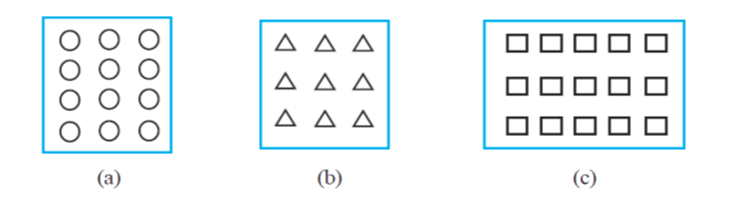### Solution

What is known?

What is unknown?

Shaded figure according to given fraction.

Reasoning:

First multiple the fraction by number of parts of given figure that’s how we will get what number of parts need to be shade.

Steps:

(i) Total number of circles in box $$(a) = 12$$

\begin{align}\frac{1}{2}\text{ of circles}&=\frac{1}{2} \times 12 \\&= 6\, \text{circles}\end{align}

So, $$6$$ circles should be shaded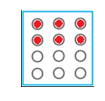(ii) Total number of triangles in box $$(b) = 9$$

\begin{align}\frac{2}{3} \text{ of triangles} &=\frac{2}{3} \times 9 \\&= 6 \,\text{triangles}\end{align}

So, $$6$$ triangles should be shaded.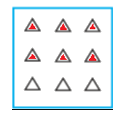iii) Total number of squares in box $$(c) = 15$$

\begin{align}\frac{3}{5} \,{\rm of }\,\times 15\, \text{squares} &= \frac{3}{5} \times 15 \\&= 3 \times 3 \\&= 9 \text{ squares}\end{align}

So, $$9$$ squares should be shaded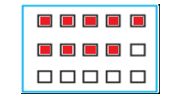## Chapter 2 Ex.2.2 Question 5

Find: -

\begin{align}\left( {\rm{a}} \right)\,\,&\frac{1}{2} \text{ of} &\\ &\qquad{\rm{i)}}\;\; 24 \\&\qquad{\rm{ ii) }}\;46\end{align}

\begin{align}\left( {\text{b}} \right)\,\,&\frac{2}{3}\text{ of }& \\ &\qquad{\rm{i)}}\;\; 18\\&\qquad{\rm{ ii) }} \;27\end{align}

\begin{align}\left( {\text{c}} \right)\,\,&\frac{3}{4} \text{of } & \\& \qquad {\rm{i)}} \;\;16\\& \qquad {\rm{ ii) }}\;36\end{align}

\begin{align}\left( {\text{d}} \right)\,\,&\frac{4}{5} \text{ of }& \\&\qquad {\rm{i)}} \;\;20 \\ &\qquad {\rm{ ii) }}\;35\end{align}

### Solution

What is known?

Fraction and whole.

What is unknown?

Part of following whole according to given fraction.

Reasoning:

Multiple whole by fraction.

Steps:

(a)

\begin{align}{{\rm{ (i) }} \;\frac{1}{2}{\rm{ of }}\;24 = \frac{1}{2} \times \frac{{24}}{1} = \frac{{24}}{2} = 12}\end{align}

\begin{align}{\rm{ (ii) }} \; \frac{1}{2}{\rm{ of }}\;46 = \frac{1}{2} \times \frac{{46}}{1} = \frac{{46}}{2} = 23\end{align}

(b)

\begin{align}{{\rm{ (i) }} \; \frac{2}{3}{\rm{ of }}\;18 = \frac{2}{3} \times \frac{{18}}{1} = 2 \times 6 = 12}\\\end{align}

\begin{align}{\rm{(ii)}} \;\frac{2}{3}{\rm{ of }}\;27 = \frac{2}{3} \times \frac{{27}}{1} = 2 \times 9 = 18\end{align}

(c)

\begin{align}\;\; {\rm{(i)}} \;\frac{3}{4}{\rm{ of }}\;16 = \frac{3}{4} \times \frac{{16}}{1} = 3 \times 4 = 12\end{align}

\begin{align}\quad {\rm{(ii)}} \; \frac{3}{4}{\rm{ of }}\;36 = \frac{3}{4} \times \frac{{36}}{1} = 3 \times 9 = 27\end{align}

(d)

\begin{align} \;\; {\rm{(i)}} \; \frac{4}{5}{\rm{ of }}\;20 = \frac{4}{5} \times \frac{{20}}{1} = 4 \times 4 = 16\end{align}

\begin{align} \;\; {\rm{(ii)}} \; \frac{4}{5}{\rm{ of }}\;35 = \frac{4}{5} \times \frac{{35}}{1} = 4 \times 7 = 28\end{align}

## Chapter 2 Ex.2.2 Question 6

Multiply and express as a mixed fraction:

(a) \begin{align}3 \times 5\frac{1}{5}\end{align}

(b) \begin{align} 5 \times 6\frac{3}{4}\end{align}

(c) \begin{align}7 \times 2\frac{1}{4}\end{align}

(d) \begin{align}4 \times 6\frac{1}{3}\end{align}

(e) \begin{align}3\frac{1}{4} \times 6\end{align}

(f) \begin{align}3\frac{2}{5} \times 8\end{align}

### Solution

What is known?

Expression.

What is unknown?

Product of given expression in mixed fraction.

Reasoning:

Covert mixed fraction into improper fraction then find the product and covert the answer into mixed fraction.

Steps:

(a)

\begin{align}3 \times 5\frac{1}{5} &= \frac{3}{1} \times \frac{{26}}{5} \1mm]&= \frac{{3 \times 26}}{5} \\&= \frac{{78}}{5} \text{( improper fraction )} \end{align} Converting\begin{align}\frac{{78}}{5}\end{align}into mixed fraction, we get \[\begin{align}\frac{{78}}{5} = 15\frac{3}{5}\end{align}

(b)

\begin{align}5 \times 6\frac{3}{4} &= \frac{5}{1} \times \frac{{27}}{4} \0.5mm]&= \frac{{5 \times 27}}{4} \\[0.5mm] &= \frac{{135}}{4}({\text{ improper fraction }})\end{align} Converting \begin{align}\frac{{135}}{4}\end{align} into mixed fraction, we get \[\begin{align}\frac{{135}}{4} = 33\frac{3}{4}\end{align}

(c)

\begin{align}7 \times 2\frac{1}{4} &= \frac{7}{1} \times \frac{9}{4} \0.5mm]&= \frac{{7 \times 9}}{4} \\[0.5mm]&= \frac{{63}}{4}({\text{ improper fraction }})\end{align} Converting \begin{align}\frac{{63}}{4}\end{align} into mixed fraction, we get \[\begin{align} \frac{{63}}{4} = 15\frac{3}{4}\end{align}

(d)

\begin{align}4 \times 6\frac{1}{3} &= \frac{4}{1} \times \frac{{19}}{3} \0.5mm]&= \frac{{4 \times 19}}{3} \\[0.5mm]&= \frac{{76}}{3}({\text{ improper fraction }})\end{align} Converting \begin{align}\frac{{76}}{3}\end{align} into mixed fraction, we get \[\begin{align}\frac{{76}}{3} = 25\frac{1}{3}\end{align}

(e)

\begin{align}3\frac{1}{4} \times 6 &= \frac{{13}}{4} \times \frac{6}{1} \0.5mm]&= \frac{{13 \times 6}}{4} \\[0.5mm]&= \frac{{78}}{4}({\text{ improper fraction }})\end{align} Converting \begin{align}\frac{{78}}{4}\end{align}into mixed fraction, we get \[\begin{align}\frac{{78}}{4} = 19\frac{1}{2}\end{align}

(f)

\begin{align}3\frac{2}{5} \times 8 &= \frac{{17}}{5} \times \frac{8}{1} \0.5mm]&= \frac{{17 \times 8}}{5} \\[0.5mm]&= \frac{{136}}{5}({\text{ improper fraction }})\end{align} Converting \begin{align}\frac{{136}}{5}\end{align} into mixed fraction, we get \[\begin{align}\frac{{136}}{5} = 27\frac{1}{5}\end{align}

## Chapter 2 Ex.2.2 Question 7

Find

\begin{align} {\rm{(a)}}\,\, &\frac{1}{2}\;{\rm{ of }} \\& \qquad ({\rm{ i }})\;\;2\frac{3}{4} \\&\qquad {\rm{ (ii) }}\;4\frac{2}{9}\end{align}

\begin{align} {\rm{(b)}}\,\, &\frac{5}{8}\;{\rm{ of }}\\ & \qquad ({\rm{ i }})\;\;3\frac{5}{6} \\ &\qquad {\rm{ (ii) }}\;9\frac{2}{3}\end{align}

### Solution

What is known?

Fractions

What is unknown?

Product of the given fractions.

Reasoning:

Covert mixed fraction into improper fraction then find the product.

Steps:

(a)

\begin{align}({\rm{i}})\;\frac{1}{2}\,{\rm{ of }}\;2\frac{3}{4}\end{align}

\begin{align}\frac{1}{2}\text{ of }2\frac{3}{4}&=\frac{1}{2}\times 2\frac{3}{4}\0.5mm]&=\frac{1}{2}\times \frac{11}{4}\\[0.5mm]&=\frac{11}{8} \\&\left( \text{improper fraction} \right)\end{align} Converting \begin{align}\frac{{11}}{8}\end{align} into mixed fraction, we get, \[\begin{align}\frac{{11}}{8} = 1\frac{3}{8}\end{align}

\begin{align} {\rm{(ii)}} \;\frac{1}{2}\;{\rm{ of }}\;4\frac{2}{9} \end{align}

\begin{align} \frac{1}{2}\;{\rm{ of }}\;4\frac{2}{9} &= \frac{1}{2} \times \frac{{38}}{9} \0.5mm]&= \frac{{19}}{9}\\&({\text{improper fraction}})\end{align} Converting \begin{align}\frac{{19}}{{9}}\end{align} into mixed fraction, we get, \[\begin{align}\frac{{19}}{9} = 2\frac{1}{9}\end{align}

(b) \begin{align}\frac{5}{8}\;\;{\rm{ of (i) }}\;3\,\frac{5}{6}\quad {\rm{ (ii) }}\;9\frac{2}{3}\end{align}

(i)

\begin{align}\;\frac{5}{8}\;{\rm{ of }}\;3\frac{5}{6} &= \frac{5}{8} \times \frac{{23}}{6} \0.5mm]&= \frac{{115}}{{48}}\\&({\text{improper fraction)}}\end{align} Converting \begin{align}\frac{{115}}{{48}}\end{align} into mixed fraction, we get, \[\begin{align}\frac{{115}}{{48}} = 2\frac{{19}}{{48}}\end{align}

(ii)

\begin{align}\frac{5}{8}\;{\rm{ of }}\;9\frac{2}{3} &= \frac{5}{8} \times \frac{{29}}{3} \0.5mm]&= \frac{{145}}{{24}}\\&({\text{improper fraction}})\end{align} Converting \begin{align}\frac{{145}}{{24}}\end{align} into mixed fraction, we get, \[\begin{align}\frac{{145}}{{24}} = 6\frac{1}{{24}}\end{align}

## Chapter 2 Ex.2.2 Question 8

Vidya and Pratap went for a picnic. Their mother gave them a water bottle that contained $$5$$ liters of water. Vidya consumed \begin{align}\frac{2}{5}\end{align} of the water. Pratap consumed the remaining water.

i) How much water did Vidya drink?

ii) What fraction of the total quantity of water did Pratap drink?

### Solution

What is known?

Total quantity of water and fraction of water consumed by Vidya.

What is unknown?

How much water did Vidya drink and what fraction of the total quantity of water did Pratap drink.

Reasoning:

Simply multiple the total quantity of water with fraction of water consumed by Vidya to find out how much she consumed. Subtract the fraction of water consumed by Vidya from total to get what fraction of water Pratap drink.

Steps:

Total quantity of water in a bottle $$=\rm{} 5$$ liters

(i) Water consumed by Vidya

\begin{align}&=\frac{2}{5} \text{of total water} \\&= \frac{2}{5} \times 5 = 2\end{align}

Thus, Vidya consumed $$2$$ liters of water.

(ii) Water consumed by Pratap

\begin{align} &= 1 - \frac{2}{5} \\&=\frac{3}{5}{\text{ of the total water}}\end{align}

Thus, \begin{align}\frac{3}{5}\end{align}of the total water consumed by Pratap.

Related Sections
Related Sections
Instant doubt clearing with Cuemath Advanced Math Program